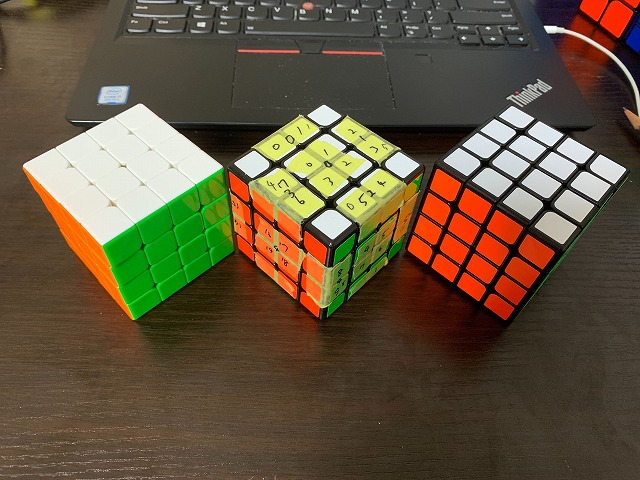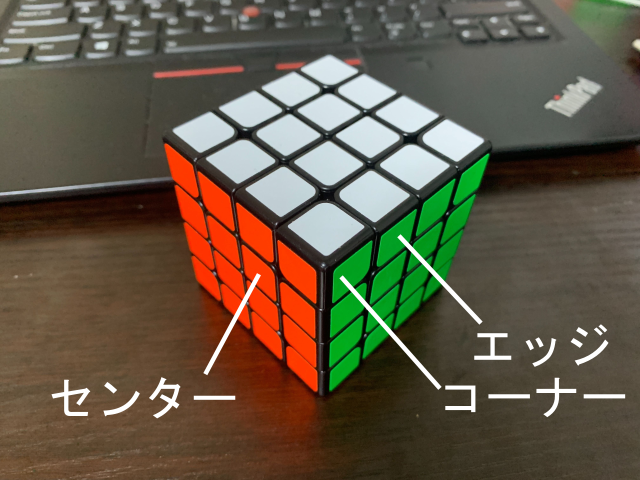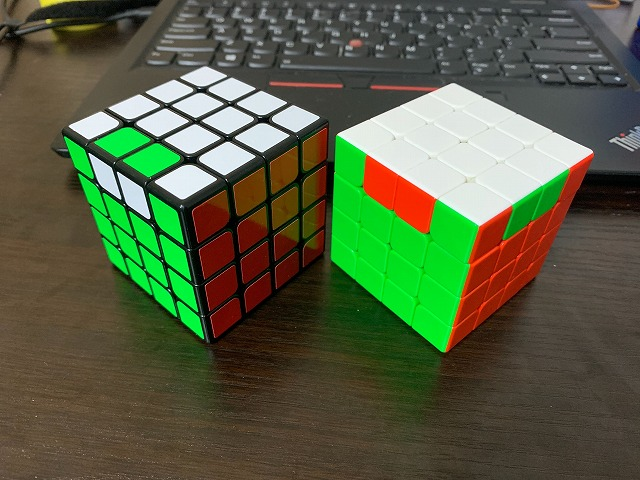# [PYTHON] Write a program to solve the 4x4x4 Rubik's Cube! 1. Overview

I am currently making a robot that solves the 4x4x4 Rubik's Cube (Rubik's Revenge) and is aiming for a world record. The biggest hurdle in making a robot was to implement an algorithm to solve a 4x4x4 cube in a realistic time and with as few steps as possible. If you look it up, you will find that there are few documents and ancestors about 4x4x4. I am writing this article, hoping that it will be one of such valuable materials. GitHub is here ** The content of this article is not complete. It may contain some inefficiencies. I will add it as needed if it is improved in the future. ** **

↓ Competition 4x4x4 Rubik's Cube and Competition 4x4x4 Rubik's Cube numbered for program production# The whole picture

This collection of articles consists of three articles in total.

2. Algorithm
3. Implementation

In this article, I will introduce the knowledge necessary to write a program to solve a 4x4x4 Rubik's cube (using a search algorithm), and briefly talk about the flow of the program.

# Reference material

I will introduce the reference materials in the order in which I find them helpful.

• https://arxiv.org/abs/1601.05744
• https://github.com/cs0x7f/TPR-4x4x4-Solver
• http://cubezzz.dyndns.org/drupal/?q=node/view/525
• http://cubezzz.dyndns.org/drupal/?q=node/view/73

The first source is the only paper I have found about 4x4x4 cubes. The second is a repository that is actually implemented using Java in a manner similar to a dissertation. This was also found as the only implementation example. The third is a post by the owner of the second repository. The fourth is a mysterious post that was found for the time being.

Here's what you need to know to read this article and how to get it.

## Rotation symbol

A symbol that objectively and accurately represents the rotation of the Rubik's cube. We recommend here as reference material. This is a description of the rotation symbol for 3x3x3, but 4x4x4 uses exactly the same symbol.

## Face name

The `` `R, L, U, D, F, B``` that appear in the rotation symbol is also used as the name of the face. For example, the F side is the front side.

## Part name

There are three types of parts on the outside of the 4x4x4 Rubik's Cube. As shown in the image.There are 3 stickers on the corners, 2 on the edges and 1 on the center.

## How to represent the position of parts

For the position of the parts, use the `` `R, L, U, D, F, B``` that appears again with the rotation symbol. Since the expression is different for edges and corners, I will introduce them separately.

### Edge

The edge is natural, but it always straddles two sides. Therefore, the edges are represented side by side by taking two faces that straddle from `` `R, L, U, D, F, B```. For example, the UF edge is the edge that straddles the U and F planes.

### corner

The corner always straddles three sides. Therefore, three faces that straddle from `` `R, L, U, D, F, B``` are taken and displayed side by side. For example, the UFR corner is a corner that straddles the U, F, and R sides.

## parity

The 4x4x4 Rubik's Cube has a unique condition called "parity". There are two types of parity, but the explanation of here is easy to understand. In addition, the explanation of PLL parity is a little difficult to understand on this site, but in short, it is a state of 2-point exchange of only edges (2 points exchange is not possible with 3x3x3 Rubik's cube). The figure shows an example of OLL parity and PLL parity. The left is OLL parity and the right is PLL parity. All the invisible sides are complete.CP, CO, EP, EO Each

• Corner Permutation
• Corner Orientation
• Edge Permutation
• Edge Orientation

That is. Each part that makes up a 4x4x4 cube can be uniquely represented by the position and orientation of the edges and corners, and the position of the center.

# Base algorithm

Solving a 4x4x4 Rubik's cube by exploration is not so easy. Anyway, the tree to be searched (the term and basic knowledge of this is summarized in the article here) is too big. Therefore, an algorithm is often used. The materials presented and my implementation are all based on an algorithm called ** Tsai's Algorithm **. Let's explain the flow. In addition, if it is written that "` X``` rotation is used, for example, in "Rotation to use", there are two types of rotations that are actually used: "` X, X''`And if it says to use` `X2`rotation, only` X2``` is actually used. I think there are words and expressions that I don't understand. I will explain each phase in detail in the next article, so please say "Hmm" here.

Phase number things to do Rotation to use
0 Bring all the center parts of R side and L side to R side or L side `R, R2, Rw, Rw2, L, L2, U, U2, Uw, Uw2, D, D2, F, F2, Fw, Fw2, B, B2`
1 1.Bring all the center parts of F side and B side to F side or B side 2.Separate high edge and low edge 3.Make the center state of R and L planes one of 12 states that can be processed in the future 4.Eliminate OLL parity `R, R2, Rw, Rw2, L, L2, U, U2, Uw2, D, D2, F, F2, Fw2, B, B2`
2 1.side(`F, R, B, L`surface)Make a "row" in the center 2.sideに位置するエッジのペアリングを行う `R2, Rw2, L2, U, U2, Uw2, D, D2, F, F2, Fw2, B, B2`
3 1.Complete 6 centers 2.Pair the remaining edges 3.Eliminate PLL parity `R2, Rw2, L2, U, U2, Uw2, D, D2, F2, Fw2, B2`
4 1.Bring the stickers that should be on the U and D sides to the U or D side 2.Eliminate EO `R, L, U, U2, D, D2, F, B`
5 Finalize `R2, L2, U, U2, D, D2, F2, B2`

# Summary

In this article, I gave a brief explanation of the knowledge required to write a program to solve a 4x4x4 Rubik's cube and an overview of how to solve a 4x4x4 Rubik's cube.